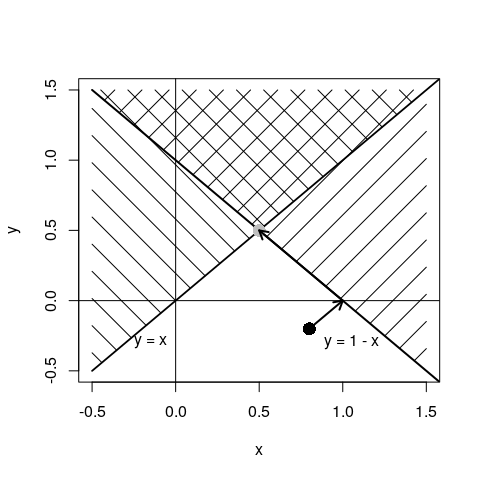# Introduction to the Successive Projection Algorithm

## Introduction

The successive projection algorithm (SPA) solves quadratic optimization problems under linear equality and inequality restrictions. That is, given a vector $$\boldsymbol{x}$$, find the vector $$\boldsymbol{x}^*$$ that minimizes the weighted Euclidian distance $(\boldsymbol{x}-\boldsymbol{x}^*)^T\boldsymbol{W}(\boldsymbol{x}-\boldsymbol{x}^*),$ subject to $\boldsymbol{Ax}^*\leq \boldsymbol{b}.$ Here, $$\boldsymbol{W}$$ is a diagonal matrix with positive weights. The system of restrictions can contain equality and/or inequality restrictions.

### Example

Suppose we have the vecor $$(x,y)=(0.8,-0.2)$$, depicted by the black dot in the figure below. Furthermore, we have the demands that

$\begin{array}{lcl} y &\geq& x\\ x &\geq& 1-y \end{array}$ The regions where $$y\geq x$$ or $$x\geq 1-y$$ are indicated by the single-shaded regions in the figure. The area where both demands are satisfied is indicated by the doubly-shaded region.To find a solution, the successive projection algorithm projects the start vector iterativelty on the borders of the convex region that is defined by the linear inequalities. In the figure this is indicated by the arrows. The solution is a point on or numerically very near the border of the allowed region.

When all weights on the diagonal of $$\boldsymbol{W}$$ are equal, projections are orthogonal, as shown in the figure. If the weights differ, the direction of projections will be scaled accordingly.

### Optimization in R

In the lintools package, all inequalities must be written in the $$\leq$$-form. So first note that the above constraints can be written as $\left(\begin{array}{cc} 1 & -1\\ 1 & 1\\ \end{array}\right) \left(\begin{array}{c} x\\y \end{array}\right) \leq \left(\begin{array}{c} 0\\ 1 \end{array}\right)$

So we formulate the problem with the lintools package as follows.

library(lintools)
x <- c(0.8,-0.2)
A <- matrix(c(1,-1,-1,-1), byrow=TRUE, nrow=2)
b <- c(0,-1)

The function project solves the problem for us. By passing neq=0 we tell project that every restriction is an inequality (setting neq>0 means that the first neq restrictions are equalities).

project(x=x,A=A,b=b,neq=0)
## $x ##  0.5 0.5 ## ##$status
##  0
##
## $eps ## NULL ## ##$iterations
##  2
##
## $duration ## user system elapsed ## 0 0 0 ## ##$objective
##  0.7615773

The result is a list with the following elements.

• x : the optimized vector
• status: A status indicator: 0 means that the algorithm converged to a solution. (1= not enough memory, 2= divergence detected, 3 = maximum nr of iterations exceeded)
• tol A measure of how far the final vector lies from the borders defined by the constaint (it is the $$L_\infty$$ distance to the valid region).
• iterations The number of iterations performed.
• duration The amount of time elapsed during optimization.
• objective This is the weighted distance between the start vector and the solution.

## Sparse problems

For problems where a great many coeffiecients need to be optimized under a large number of restrictions, it is possible to forumate the restrictions in sparse format.

In the lintools package, the row-column-coefficient format is used. That is, in stead of defining the full matrix $$\boldsymbol{A}$$ as in the previous example, we set up a data.frame with columns

• row : the row number
• column: the column number
• coef : the coefficient

Of course, only non-zero coefficients need to be listed.

As a -rather simple- example, we define the same problem as above, but now in a sparse manner.

A <- data.frame(
row = c(1,1,2,2)
,col = c(1,2,1,1)
,coef = c(1,-1,-1,-1)
)
b <- c(0,-1)
x <- c(0.8,-0.2)

Solving is done with the sparse_project function.

sparse_project(x, A=A, b=b, neq=0)
## $x ##  0.5 0.5 ## ##$status
##  0
##
## $eps ## NULL ## ##$iterations
##  3
##
## $duration ## user system elapsed ## 0 0 0 ## ##$objective
##  0.7615773

We have been able to solve problems with up to $$\sim$$ 6 milion variables and hundreds of thousands of linear (in)equality restrictions with the algorithm as implemented in this package.

### Reusing sparsely defined restrictions

The sparse_project function performs the following steps:

1. It creates a particular sparse representation of the restrictions
2. It solves the minimization problem
3. It gathers results and returns them to the user.

Step 1 takes a little bit of time (not much) but if you need to do a lot of optimizations it may pay to do it once and reuse the representation. This can be done as follows, using the same definition of sparse constraints as in the previous subsection.

First, create an object of class sparse_constraints.

sc <- sparse_constraints(A,b,neq=0)

Now, using its project method, we can optimize from multiple starting points, for example:

sc$project(x=c(0.8,-0.2)) ##$x
##  0.5 0.5
##
## $status ##  0 ## ##$eps
## NULL
##
## $iterations ##  3 ## ##$duration
##    user  system elapsed
##       0       0       0
##
## $objective ##  0.7615773 # the same problem, but with differing weights sc$project(x=c(0.8,-0.2),w=c(1,10))
## $x ##  0.5040358 0.4959642 ## ##$status
##  0
##
## $eps ## NULL ## ##$iterations
##  26
##
## $duration ## user system elapsed ## 0 0 0 ## ##$objective
##  2.220643

## Some words on the rspa package

Users of the rspa package will no doubt recognize the algorithm and the sparse_constraints object. We chose to separate the functionality from rspa to be able to reuse the successive projection algorithm for multiple purposes, without depending on the editrules package. In the future, rspa will depend on lintools with guaranteed backward compatibility.